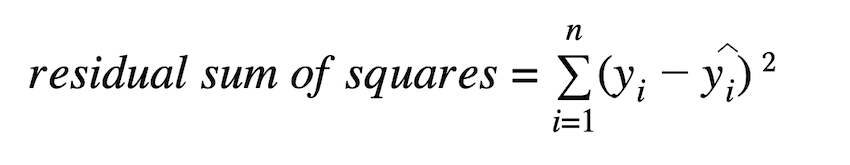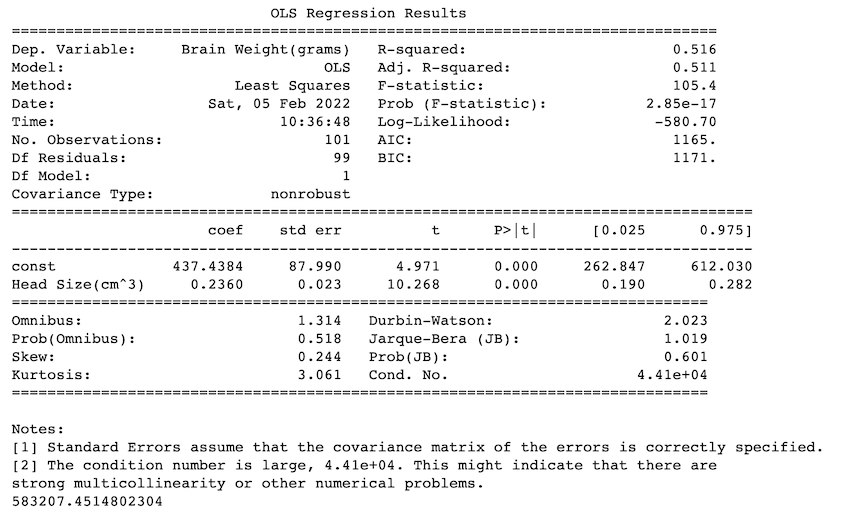# How to Calculate Residual Sum of Squares in Python

• Last Updated : 21 Feb, 2022

The residual sum of squares (RSS) calculates the degree of variance in a regression model. It estimates the level of error in the model’s prediction. The smaller the residual sum of squares, the better your model fits your data; the larger the residual sum of squares, the worse. It is the sum of squares of the observed data minus the predicted data.

#### Formula:## Method 1: Using Its Base Formula

In this approach, we divide the datasets into independent variables and dependent variables. we import sklearn.linear_model.LinearRegression(). we fit the data in it and then carry out predictions using predict() method. as the dataset only contains 100 rows train test split is not necessary.

## Python

 `# import packages``import` `pandas as pd``import` `numpy as np``from` `sklearn.linear_model ``import` `LinearRegression`` ` ` ` `# reading csv file as pandas dataframe``data ``=` `pd.read_csv(``'headbrain2.csv'``)`` ` `# independent variable``X ``=` `data[[``'Head Size(cm^3)'``]]`` ` `# output variable (dependent)``y ``=` `data[``'Brain Weight(grams)'``]`` ` `# using the linear regression model``model ``=` `LinearRegression()`` ` `# fitting the data``model.fit(X, y)`` ` `# predicting values``y_pred ``=` `model.predict(X)``df ``=` `pd.DataFrame({``'Actual'``: y, ``'Predicted'``:``y_pred})`` ` `print``(``' residual sum of squares is : '``+` `str``(np.``sum``(np.square(df[``'Predicted'``] ``-` `df[``'Actual'``]))))`

Output:

` residual sum of squares is : 583207.4514802304`

## Method 2: Using statsmodel.api

In this approach, we import the statsmodel.api. After reading the datasets, similar to the previous approach we separate independent and dependent features. We fit them in sm.OLS() regression model. This model has a summary method that gives the summary of all metrics and regression results. model.ssr gives us the value of the residual sum of squares(RSS). We can see that the value we derived from the previous approach is the same as model.ssr value.

## Python

 `# import packages``import` `pandas as pd``import` `numpy as np``import` `statsmodels.api as sm`` ` `# reading csv file as pandas dataframe``data ``=` `pd.read_csv(``'headbrain2.csv'``)`` ` `# independent variable``x ``=` `data[``'Head Size(cm^3)'``]`` ` `# output variable (dependent)``y ``=` `data[``'Brain Weight(grams)'``]`` ` `# adding constant``x ``=` `sm.add_constant(x)`` ` `#fit linear regression model``model ``=` `sm.OLS(y, x).fit()`` ` `#display model summary``print``(model.summary())`` ` `# residual sum of squares``print``(model.ssr)`

Output:

`583207.4514802304`My Personal Notes arrow_drop_up